Question

Implement a C++ map to store data in a file, such as any .dat or .txt file filled with data. The data should be loaded to map from the data file.

#include<iostream> //for cout
#include<fstream> //for ifstream
#include<map> //for map
#include<iterator> //for iterator
using namespace std;

//main function
int main()
{
map<int,int> m; //declared variable of map
ifstream in; //declared in stream
in.open("input.txt"); //opens input.txt file
int x,y;
if(in==NULL){ //if file fails to open then prints message and terminates program
cout<<"Error occurred while opening file";
return -1;
}
while(in>>x>>y) //reads values into x and y
{
m.insert(pair<int,int>(x,y)); //inserts values accordingly into map
}
//creates and iterator for map
map<int,int>::iterator it = m.begin();
while(it!=m.end()){ //iterates till end of map and prints the values in map
cout<<it->first<<" "<<it->second<<endl;
it++;
}

return 0; //terminates the program
}

//output screenshot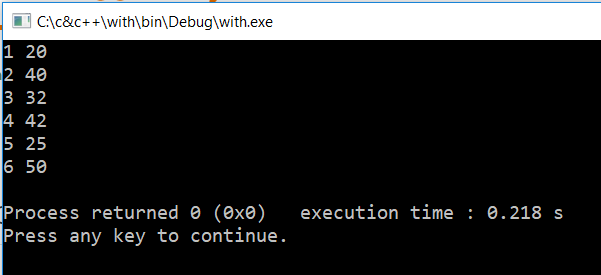//input.txt screenshot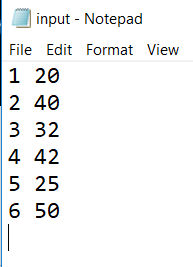//any query, post in the comment section

#### Earn Coins

Coins can be redeemed for fabulous gifts.

Similar Homework Help Questions
• ### create a program that sum value from a txt file in c++,the data in the txt...

create a program that sum value from a txt file in c++,the data in the txt file are in columns

• ### C++ Problem: Power Plant Data. The data file power1.dat contains a power plant output in megawatts...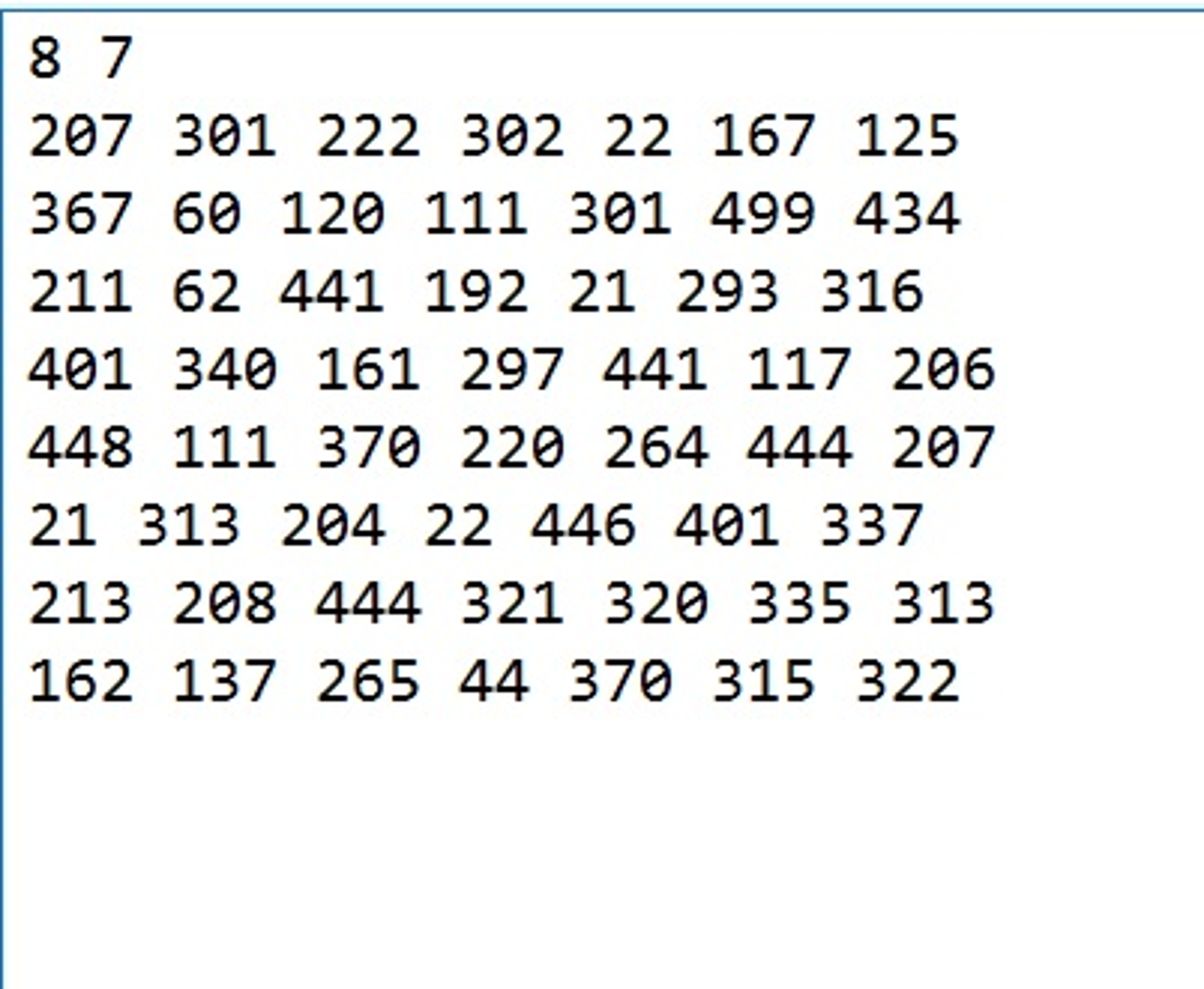C++ Problem: Power Plant Data. The data file power1.dat contains a power plant output in megawatts over a period of 10 weeks. Each row of data contains 7 floating-point numbers that represent 1 week's data. In developing the following programs, use symbolic constants NROWS and NCOLS to represent the number of rows and columns in the array used to store the data. Write a function to compute and return the average value in a two-dimensional vector of type double. Assume...

• ### Using java: Store the files in .txt format and use the readByte() and writeByte() methods. File...

Using java: Store the files in .txt format and use the readByte() and writeByte() methods. File encryption is the science of writing the contents of a file in a secret code. Write an encryption program that works like a filter, reading the contents of one file, modifying the data into a code, and then writing the coded contents out to a second file. The second file will be a version of the first file, but written in a secret code....

• ### Using C++ coin1.txt ..-'''''-.. .' ___ '. / .'\ `\ \ ; /, ( | ; ; /_ '._ / ; | |- '._`) | ; '-...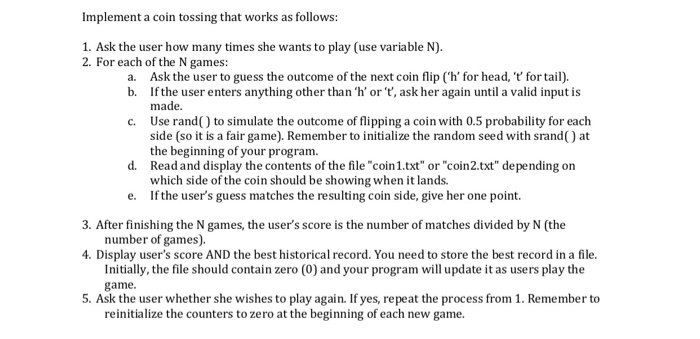Using C++ coin1.txt ..-'''''-.. .' ___ '. / .'\ `\ \ ; /, ( | ; ; /_ '._ / ; | |- '._`) | ; '-;-' \ ; ; '''' / \ ; \ '.__..-' / '._ QUARTER _.' ''----''' coin2.txt ..-'''''-.. .' T E X A S '. / __ \ ; | |_ ; ; ___ | `~\ ; | \ ) | ; \/~ / ; ; '''' \ / ; \ \_\ / '._ 2...

• ### Assume that an array A is given to you in a txt file. You should read...

Assume that an array A is given to you in a txt file. You should read it. If you think this is a time-dependent data, print the number of local minimums in the array to a txt file. Local minimum means the element which is less than the previous element and the following element. Please do not use any additional library. For example, if A=[3, 2, 9, 8, 7, 8, 6], the code should print 2 and 7. (Local minimums...

• ### Write a C++ segment of code in main () that reads a file called "numbers txt"....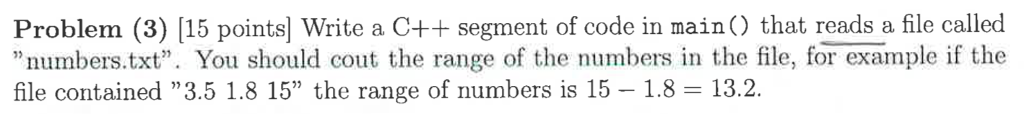Write a C++ segment of code in main () that reads a file called "numbers txt". You should cout the range of the numbers in the file, for example if the file contained "3.5 1.8 15" the range of numbers is 15 - 1.8 = 13.2.

• ### Python Basic Lists, File IO, Exception Handling 1. Call createTable() to: Read in the data from...

Python Basic Lists, File IO, Exception Handling 1. Call createTable() to: Read in the data from each of the input files (in order from scores1.txt to scores5.txt) and store the data in table. The table is a list of lists, where the scores of each input file is a column of data of the table. By the end of the function, the table should have 5 columns of numbers, each column is from one input file. Here is the first...

• ### Implement MERGE-SORT() algorithm that reads from a file named “inputHW02.txt” a list of double numbers (max...

Implement MERGE-SORT() algorithm that reads from a file named “inputHW02.txt” a list of double numbers (max = 3,000,000 numbers), sorts those numbers and indicates time consumption. This programming question will address the advantage of using iteration loops over recursive calls as well as using INSERTION-SORT() as a procedure in MERGESORT(). Your program must perform the following actions: 1. Opens the given file name and reads all double numbers. For simplicity, we assume this file only contains numbers and nothing else....

• ### Write a C++ program that will read a large .txt file and will find the highest...

Write a C++ program that will read a large .txt file and will find the highest and lowest voltage, along with the associated time and ampere. The text file is large 1,000,000 rows of data, so speed is a factor. Per some of my own research it may be best to use mmap (If I have the fastest program I will receive a significant amount of extra credit)? Someone also told me that binary can be fast, but I have...

• ### write a code on .C file Problem Write a C program to implement a banking application...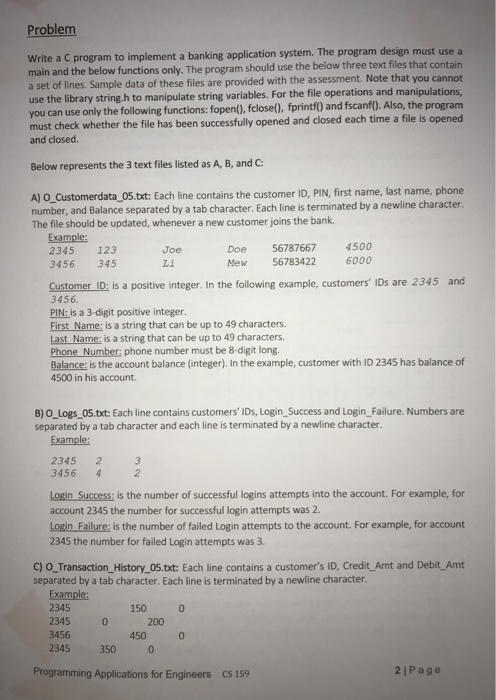write a code on .C file Problem Write a C program to implement a banking application system. The program design must use a main and the below functions only. The program should use the below three text files that contain a set of lines. Sample data of these files are provided with the assessment. Note that you cannot use the library string.h to manipulate string variables. For the file operations and manipulations, you can use only the following functions: fopen(),...